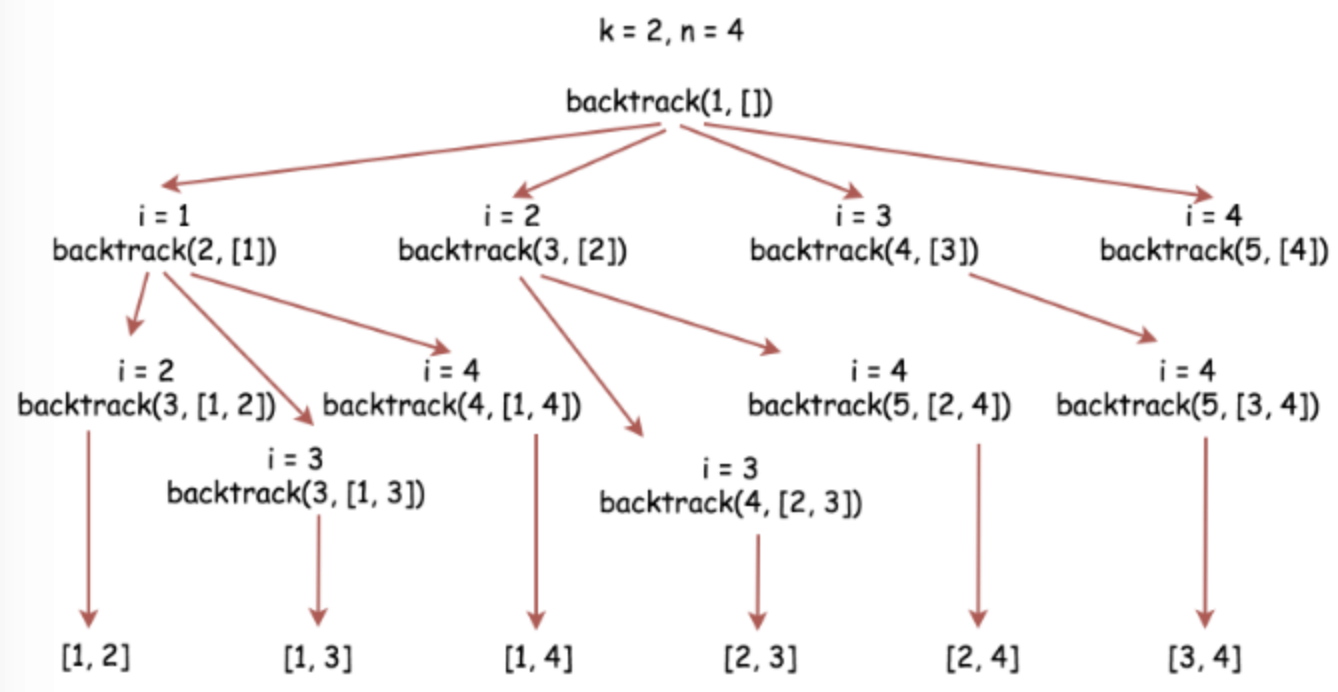# 77. Combinations

Reference: LeetCode EPI 15.6
Difficulty: Medium

## Problem

Given two integers $n$ and $k$, return all possible combinations of $k$ numbers out of $1\ldots n$.

Example:

## Analysis

### BacktrackingOne brute-force approach is to compute all subsets of ${1,2,\ldots, n}$, and then restrict the result to subsets of size $k$. It computes all subsets though, which takes $O(n 2^n)$ time regardless of $k$.

Take ${1, 2, 3, 4}$ and $k = 3$ as an example.

We use a more focused approach. We make use of case analysis. There are two possibilities for a subset—it does not contains $1$, or it does contains $1$. In the first case, we return all subsets of size $k$ of ${2, 3, 4}$; in the second case, we compute all $(k - 1)$ sized subsets of ${2, 3, 4}$.

See the example above. We can handle the impossible cases by comparing the number of remaining choices with the $(k - \text{number of picked elements})$. For example, when d = 1, i = 3, we call (n, k, d = i + 1 = 4), the remaining number of elements equals n - i + 1 = 4 - 4 + 1 = 1, which is less than k - pickedList.size() = 3 - 1 = 2.

In the base case above, we should return right away without updating the result list. However, there exists another base case when k == pickedList.size(). In this case, we should copy the current list and put it into the result list.

Note: The idea is not difficult, but how we implement it is challenging since we should consider what parameters we need in the recursive function.

• In my version, n and k are not changing.

Time: $O(k C^k_N)$, where $C^k_N = \frac{N!}{(N-k)!k!}$.
Space: $O(k C^k_N)$

CommentJunhao Wang
a software engineering cat